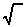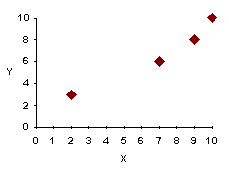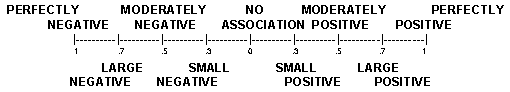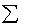previous lesson

Pearson's r

INTERVAL DATA: Association & Inference

Pearson's r: r = N(XY) - X(Y)
-----------------[N(X2) - (X)2 ][N(Y2) - (Y)2 ]

Where: X = one variable

Y = other variable

N = total in sample

Assumptions: linear relationship; homoscedasticity

Example:
 Person Height X Income Y A 10 10 B 8 9 C 6 7 D 3 2

Q: What is the association between height and income?

A: First check assumptions by making a scatter diagram.This is a linear relationship, so it also meets the assumption for homoscedasticity.
 Person Height X X2 Income Y Y2 XY A 10 100 10 100 100 B 8 64 9 81 72 C 6 36 7 49 42 D 3 9 2 4 6 N=4 27 209 28 234 220

r = 4(220) - 27(20) = ----------------------------124 =  --------------------- .97
---[4(209) - (27)2 ][4(234) - (20)2 ] ----------

Interpretation: Use the scale: "There is a ______ association between (variable 1) and (variable 2)."For r2: Convert to a percent and include in the statement "_____% of the variance in (variable 1) can be explained by (variable 2)." or vice versa. For 1 - r2: Convert to a percent and include in the statement, "_____% of the variance in (variable 1) cannot be explained by (variable 2)." or vice versa.

Interpretation of the example above:

Large positive relationship between height and income.

94% of variance in height can be explained by income.

6% of variance in height cannot be explained by income.

94% reduction in error when predicting height from income.

B. Test of significance for r and partial r

Test of significance for r:

1. Compute r and |r|.

2. d.f. = N - 2

3. Look up p-value of |r| in table in appendix.

Assumptions: 1) linear relationship

2) homoscedasticity

3) normal distribution of both variables in the whole population. Assume a normal distribution in this class because computation of it is beyond the scope of this course.

Example:
 Person Height X Income Y A 10 10 B 8 9 C 6 7 D 3 2

Q: Can this association be generalized to the whole population?

A: r = .97; d.f. = N - 2 = 4 - 2 = 2; |r| = .97

4. Check to see if this is a one-tailed or a two-tailed test. In this question there is no hypothesis, so do the two-tailed test. The table looks something like this:

Two-tailed test

d.f. p >.05 p = .05 ----------p = .01

1 : :

2 ----------------.950 --------------.990

3 : :

r =.97 So yes, one can generalize this association to the whole population.

C. Multivariate Association : Partial r

Partial r (r 12.3) = r12 - (r13)(r23)

(divided by)______________________________
-----------------------[1 - (r13)2 ][1 - (r23)2 ]

Where:
r12 = Pearson's r for variables 1 & 2.

r13 = " r " " 1 & 3.

r23 = " r " " 2 & 3.

Assumptions: linear relationship; homoscedasticity

Example:
 Person Education #1 Income #2 Age #3 A 10 10 5 B 8 9 4 C 6 7 3 D 3 2 2 E 1 1

Q: What is the association between education and income with age held constant?

A: Variable #3 will always be the one held constant. Call education variable #1 and income variable #2. Make scatter diagrams to check if assumptions are met. (In this example it is a stretch

to imagine a linear relationships, but proceed as if

they did.)

Recall: r = N(XY) - X(Y)

(divided by)___________________________________________
-----------[N(X2) - (X)2 ][N(Y2) - (Y)2 ]

Education (#1) Income (#2)
 Person Education #1 X2 Income #2 Y2 XY A 10 100 1 1 10 B 5 25 3 9 15 C 4 16 4 16 16 D 2 4 5 25 10 E 1 1 10 100 10 N=5 22 146 23 151 61

r12 = 5(61) - 22(23) = -.85
-----[5(146) - (22)2 ][5(151) - (23)2 ]
 Person Education #1 X2 Age#3 Y2 XY A 10 100 5 25 50 B 5 25 4 16 20 C 4 16 3 9 12 D 2 4 2 4 4 E 1 1 1 1 1 N=5 22 146 15 55 87

r13 = 5(87) - 22(15) = .95
------[5(146) - (22)2 ][5(55) - (15)2 ]

Income (#2) Age (#3)
 Person Income #2 X2 Age #3 Y2 XY A 1 1 5 25 5 B 3 9 4 16 12 C 4 16 3 9 12 D 5 25 2 4 10 E 10 100 1 1 10 N=5 23 151 15 55 49

r23 = 5(49) - 23(15) --------------------------------r23 = -.94
------[5(151) - (23)2 ][5(55) - (15)2 ]

r12.3 = (-.85) - (+.95)(-.94) =[1 - (+.95)2 ][1 - (-.94)2 ]

(-.85) - (-.893)[1 - (+.9025)][1 - (+.8836)]

r12.3 = +.043 =[.0975][.1164]

043 =.011349

.043 = + .40
.1065

Interpretation: same as for r but add 3rd variable which is held constant.

Example: r12.3 = .40, r12.32 = (.40)2 = .16, 1 - r12.32 = 1 - .16 = .84

Moderate association between education and income with age held constant.

16% of variance in education can be explained by income (or vice versa) with age held constant.

94% of variance in education cannot be explained by income (or vice versa) with age held constant.

16% reduction in error when predicting education from income (or vice versa) with age held constant.